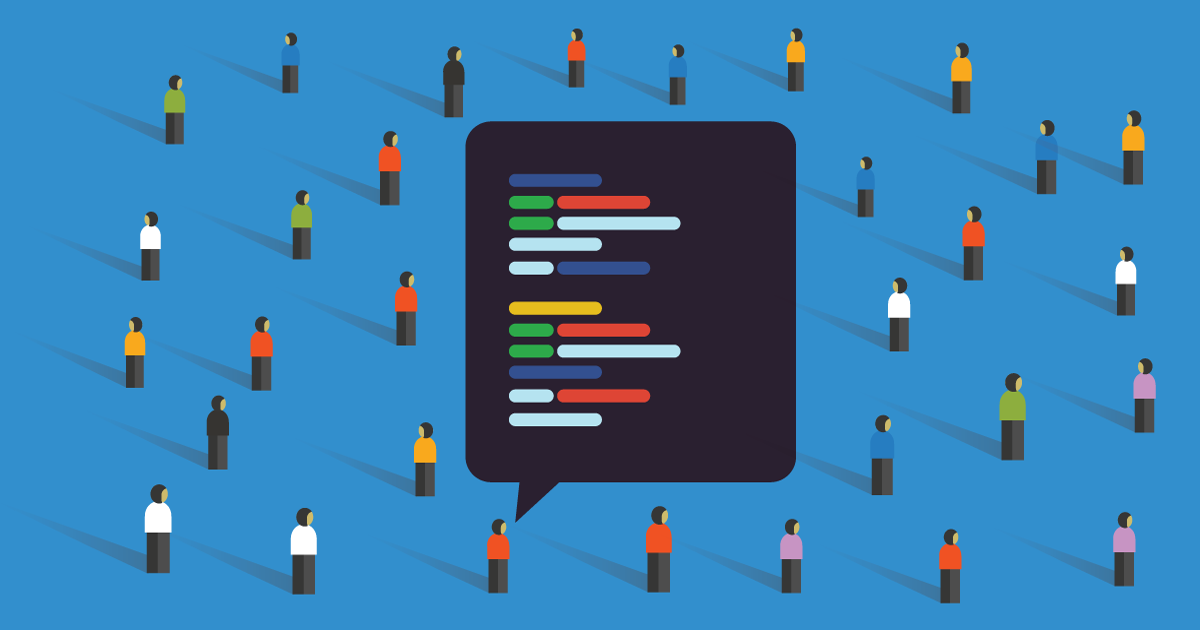Yesterday I mentioned that Haskell CSS Syntax uses a seperate module to parse any floating point numbers.

Decoding numbers involves converting to the computer's native base from our of base 10. For integers this is a simple multiply and add, as was implemented by Haskell CSS Syntax.

But for floating point we still have the same issue. "Scientific Notation" is coefficient*10^exponent, whereas computers use coefficient*2^exponent. So how does this work?

1/?

Haskell CSS Syntax yields to my code either unbounded Integers or Scientific values at it's convenient, both of which I convert to CPU-native Floats. The Scientific in turn stores a fixed-bitsize base 10 exponent and an unfixed-bitsize coefficient.

To perform the conversion for small enough exponents Scientific consults a lookup table to decide what it should multiply (for positive exponents) or take the remainder (for negative) by the coefficient.

2/?

The lookup table is constructed to start with 1 & 10, and followed by `table[i, i+1] = [x*x, 10*x*x] where x = table[i/2]`. You can do math directly on a Scientific value, but it may not be secure or performant.

If the coefficient is too large for that table (> 324) it may compute the additional exponent. Or more optimally it'll first check whether it's outside the exponents which are representable in Float's bitsize. Both approaches are implemented.

3/4?

Converting from a real number to a Scientific ammounts to long division, with some logic to prevent repeating digits from becoming infinite loops. Converting to/from text is easy as a Scientific hasn't yet been fully converted to base 2.

For an unfixed-bitsize integer it uses Haskell's builtin Integer type, which may be implemented GHC either as bindings to LibGMP or in pure Haskell. In which case it does most it's work by iterating over each fixed-bitsize "digit" in the number.

4/4 Fin.

Tomorrow I'll discuss some more of the parses I use in Rhapsode. Because personally I don't find it all that interesting to write parsers myself.

I'll discuss XML then HTML then URLs. Then consider what next...For people who care about, support, or build Free, Libre, and Open Source Software (FLOSS).